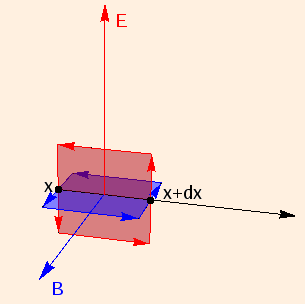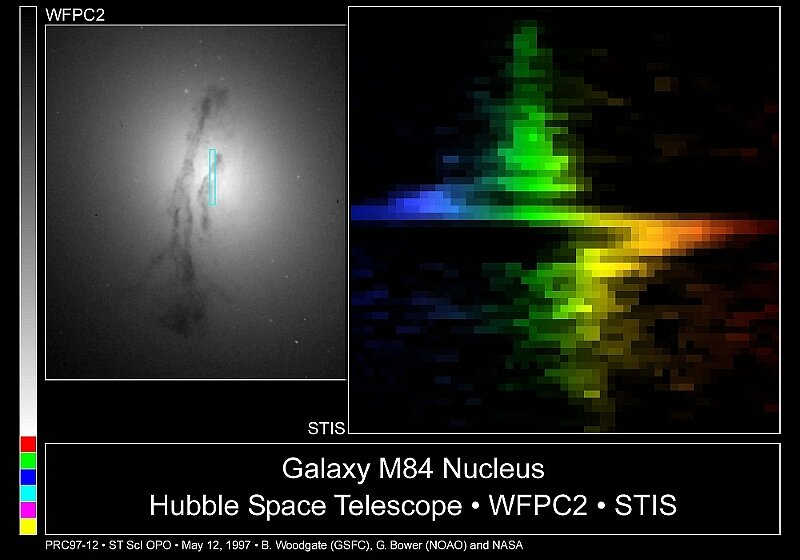### Electromagnetic Waves

1. Maxwell's correction to Ampere's law for time varying electric fields is:
closed loop B ⋅ ds = μ (I + ε dΦE/dt).
2. Taking this with Faraday's law, we see that time-varying electric fields produce magnetics fields and vice versa.

Given that such electromagnetic fields propagate energy in the direction of E ⊗ B, we examine an instant when E is along the y axis. For propagation along the x axis, B will be along the z axis. Applying Faraday's law to a loop of height dy and width dx normal to B:we have

E(x + dx) * dy - E(x) * dy = - d(dx * dy * B)/dt
which in the limit dx → 0 becomes
dE/dx = - dB/dt.
Applying Ampere's law (with I = 0) to a loop of height dz and width dx normal to E gives us
B(x) * dz - B(x + dx) * dz = μ ε d(dx * dz * E)/dt
which in the same limit becomes
dB/dx = - μ ε dE/dt.
Differentiating the first equation with respect to x and the second with respect to t and equating the results gives us the electromagnetic wave equation for E:
d2E/dx2 = d2E/dt2 / c2
with propagation velocity
c = 1 / √(μ ε) = 2.998 * 108 m/s = the speed of light.
Doing the reverse gives us the (same) wave equation for B.
3. Substituting E(x,t) = E cos (k x - ω t) and B(x,t) = B cos (k x - ω t) into dE/dx = - dB/dt, we find that the amplitudes are related by
E / B = ω / k = c.
4. The various types of electromagnetic radiation differ only by their wavelength:

radio> 1 m< 3 * 108< 1.24 * 10-6low-energy atomic or molecular motions
microwave> .1 mm< 3 * 1012< .0124rigid molecular motions
infrared> 7000 Angstroms< 4.3 * 1014< 1.78molecular bond motions
visible light> 4000 Angstroms< 7.5 * 1014< 3.1atomic electron transitions
ultraviolet> 50 Angstroms< 6 * 1016< 248atomic electron transitions
x-rays> .03 Angstroms< 1020< 414 Kelectron transitions in heavy atoms
gamma rays< .03 Angstroms> 1020> 414 Knuclear decays

Electromagnetic waves also undergo Doppler shifting, as shown here:5. The Poynting vector is
S = E ⊗ B / μ,
with units of W / m2. Since the integral of cos2 over one wavelength is 1/2, the intensity is
<S> = E2 / (2 μ c)
= c <u>
with u equal to the sum of the electric and magnetic energy densities:
ε E2 / 2 + B2 / (2 μ) = E2 / (2 μ c2) + (E / c)2 / (2 μ)
= E2 / (μ c2)
("<>" denotes the average over one wavelength, which introduces the factor of 1/2).

This implies that the electromagnetic field exerts a pressure

P = S / c
(under conditions of perfect absorption). Pressure is measured in Pascals: 1 Pa ≡ 1 N / m2.
Note that the units of pressure are identical to those of energy density.
6. The electromagnetic momentum density p = S / c2. Conservation of momentum implies that under conditions of perfect reflection, the pressure
P = 2 S / c.

 (prev) (top) (next)